# Two-dimensional manifold of bounded curvature

(diff) ← Older revision | Latest revision (diff) | Newer revision → (diff)

A metric space which is a two-dimensional manifold with a metric, for which analogues of the concepts of two-dimensional Riemannian geometry such as the length and the total curvature of a curve, the area and the total Gaussian curvature of a set have been defined.

Special cases of two-dimensional manifolds of bounded curvature are two-dimensional Riemannian spaces and polyhedral surfaces in three-dimensional Euclidean space. In the general case the class of two-dimensional manifolds of bounded curvature may be regarded as the closure of the class of two-dimensional Riemannian manifolds with respect to an appropriate limit process.

Letbe a two-dimensional Riemannian manifold, let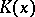be the Gaussian curvature ofat a pointand letbe the area of a set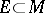; then the total curvature ofwill be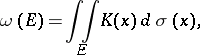its total absolute curvature will beand the positive part of the total curvature ofwill bewhere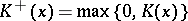. Ifandare two points in a Riemannian space, let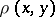be the lower bound of the lengths of the curves onwhich connect the pointsand. The functionis an internal metric; it is known as the natural metric of.

Letbe an arbitrary two-dimensional manifold with metric. One says that the metricis Riemannian if the manifoldwith the metricis isometric to some two-dimensional Riemannian space with its natural metric.

A two-dimensional manifoldwith a metricis a two-dimensional manifold of bounded curvature if the following condition is met: There exists a sequence of Riemannian metrics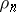,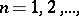defined on, such that for any compact set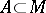one has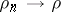uniformly (i.e. the functionsuniformly converge to the functionon the set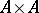) and the sequence,is bounded; here,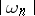is the total absolute curvature of the Riemannian metric. Two-dimensional manifolds of bounded curvature can be defined axiomatically.

The sufficient conditions in the definition of a two-dimensional manifold of bounded curvature given above may be partially weakened. Namely, a two-dimensional manifoldwith a metricwill be a two-dimensional manifold of bounded curvature if for any of its points it is possible to determine neighbourhoodsand, where, and a sequence of Riemannian metrics,defined on, such thatuniformly onand the sequenceis bounded.

For any two-dimensional manifold of bounded curvature there are defined totally-additive set functions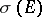and, viz., the area and the curvature of a set, respectively. In contrast to the Riemannian case,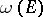need not be absolutely continuous with respect to. For two-dimensional manifolds of bounded curvature the concept of the rotation of a curve is also defined; it is the analogue of the concept of the total geodesic curvature of a curve.

Any convex surface in three-dimensional Euclidean space is a two-dimensional manifold of bounded curvature. In such a case the total curvature of a set is always non-negative.

Two-dimensional manifolds of bounded curvature can have singularities like conical points(for such pointsis non-zero), edges, borders with a cylindrical base, etc.

How to Cite This Entry:
Two-dimensional manifold of bounded curvature. Encyclopedia of Mathematics. URL: http://encyclopediaofmath.org/index.php?title=Two-dimensional_manifold_of_bounded_curvature&oldid=14396
This article was adapted from an original article by Yu.G. Reshetnyak (originator), which appeared in Encyclopedia of Mathematics - ISBN 1402006098. See original article